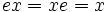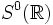# Cyclic group:Z2

(Redirected from Cyclic group of order two)
View a complete list of particular groups (this is a very huge list!)[SHOW MORE]

## Definition

### Verbal definition

The cyclic group of order 2 is defined as the unique group of order two. Explicitly it can be described as a group with two elements, say$e$ and$x$ such that$ex = xe = x$ and$e^2 = x^2 = e$. It can also be viewed as:

• The quotient group of the group of integers by the subgroup of even integers
• The multiplicative group comprising$1$ and$-1$ (in this context it is also termed the sign group)
• The additive group of the field of two elements.
• Bits under the XOR operation
• The symmetric group on two elements. In particular, it is a symmetric group on finite set and symmetric group of prime degree.
• The general linear group$GL(1,3)$ (or equivalently, the multiplicative group of the field of order 3)
• The multiplicative group of the ring$\mathbb{Z}/4\mathbb{Z}$
• The group of units in$\mathbb{Z}$
• The group$S^0(\R)$ viz., the group of vectors in$\R^1$ of unit length

This group is denoted as$C_2$,$\mathbb{Z}_2$ and sometimes as$\mathbb{Z}/2\mathbb{Z}$.

### Multiplication table

Element$e$ (identity element)$x$ (non-identity element)$e$$e$$x$$x$$x$$e$

## Arithmetic functions

### Basic arithmetic functions

Want to compare and contrast arithmetic function values with other groups of the same order? Check out groups of order 2#Arithmetic functions
Function Value Similar groups Explanation for function value
underlying prime of p-group 2
order (number of elements, equivalently, cardinality or size of underlying set) 2 groups with same order
prime-base logarithm of order 1 groups with same prime-base logarithm of order
max-length of a group 1 max-length of a group equals prime-base logarithm of order for group of prime power order
chief length 1 chief length equals prime-base logarithm of order for group of prime power order
composition length 1 composition length equals prime-base logarithm of order for group of prime power order
exponent of a group 2 groups with same order and exponent of a group | groups with same prime-base logarithm of order and exponent of a group | groups with same exponent of a group
prime-base logarithm of exponent 1 groups with same order and prime-base logarithm of exponent | groups with same prime-base logarithm of order and prime-base logarithm of exponent | groups with same prime-base logarithm of exponent
Frattini length 1 groups with same order and Frattini length | groups with same prime-base logarithm of order and Frattini length | groups with same Frattini length Frattini length equals prime-base logarithm of exponent for abelian group of prime power order
minimum size of generating set 1 groups with same order and minimum size of generating set | groups with same prime-base logarithm of order and minimum size of generating set | groups with same minimum size of generating set
subgroup rank of a group 1 groups with same order and subgroup rank of a group | groups with same prime-base logarithm of order and subgroup rank of a group | groups with same subgroup rank of a group same as minimum size of generating set since it is an abelian group of prime power order
rank of a p-group 1 groups with same order and rank of a p-group | groups with same prime-base logarithm of order and rank of a p-group | groups with same rank of a p-group same as minimum size of generating set since it is an abelian group of prime power order
normal rank of a p-group 1 groups with same order and normal rank of a p-group | groups with same prime-base logarithm of order and normal rank of a p-group | groups with same normal rank of a p-group same as minimum size of generating set since it is an abelian group of prime power order
characteristic rank of a p-group 1 groups with same order and characteristic rank of a p-group | groups with same prime-base logarithm of order and characteristic rank of a p-group | groups with same characteristic rank of a p-group same as minimum size of generating set since it is an abelian group of prime power order
nilpotency class 1 The group is a nontrivial abelian group
derived length 1 The group is a nontrivial abelian group
Fitting length 1 The group is a nontrivial abelian group

-

### Arithmetic functions of a counting nature

Function Value Similar groups Explanation for function value
number of conjugacy classes 2 groups with same order and number of conjugacy classes | groups with same number of conjugacy classes The group is abelian, so every element is its own conjugacy class. It is the only finite group with exactly two conjugacy classes. There are, however, infinite groups with exactly two conjugacy classes; see group with two conjugacy classes.
number of subgroups 2 groups with same order and number of subgroups | groups with same number of subgroups The group is a group of prime order, hence its only subgroups are itself and the trivial subgroup. Groups of prime order are the only groups with exactly two subgroups.
number of conjugacy classes of subgroups 2 groups with same order and number of conjugacy classes of subgroups | groups with same number of conjugacy classes of subgroups The group is a group of prime order, hence its only subgroups are itself and the trivial subgroup, both of which are normal and hence in different conjugacy classes. Groups of prime order are the only groups with exactly two subgroups.
number of equivalence classes under real conjugacy 2 groups with same order and number of equivalence classes under real conjugacy | groups with same number of equivalence classes under real conjugacy
number of equivalence classes under rational conjugacy 2 groups with same order and number of equivalence classes under rational conjugacy | groups with same number of equivalence classes under rational conjugacy

## Group properties

### Basic properties

Property Satisfied? Explanation
group of prime order Yes
simple group Yes
cyclic group Yes
abelian group Yes Follows from cyclic implies abelian
nilpotent group Yes Follows from abelian implies nilpotent
solvable group Yes Follows from nilpotent implies solvable
supersolvable group Yes Follows from being a finitely generated abelian group.

### Other properties

Property Satisfied? Explanation
rational-representation group Yes
rational group Yes
ambivalent group Yes
strongly ambivalent group Yes
T-group Yes
Schur-trivial group Yes

## Endomorphisms

### Automorphisms

The cyclic group of order two has no nontrivial automorphisms. In fact, it is the only nontrivial group with the property of having no nontrivial automorphisms. Further information: trivial automorphism group implies trivial or order two

### Endomorphisms

The cyclic group of order two admits two endomorphisms: the identity map and the trivial map (the map sending both elements to the identity element).

## Subgroup structure

### Subgroups

There are only two subgroups: the trivial subgroup and the whole group. Most of the nice subgroup properties are true for both.

### Quotients

There are only two quotients: itself and the trivial quotient.

## Supergroups

Further information: supergroups of cyclic group:Z2

### Occurrence as a subgroup

The cyclic group of order 2 occurs as a subgroup in many groups. In general, any group of even order contains a cyclic subgroup of order 2 (this follows from Cauchy's theorem, which is a corollary of Sylow's theorem, though it can also be proved by a direct counting argument). Elements of order 2, which are generators for cyclic groups of order 2, are termed involutions.

### Occurrence as a normal subgroup

The cyclic group of order 2 may occur as a normal subgroup in some groups. Examples are the general linear group or special linear group over a field whose characteristic is not 2. This is the group comprising the identity and negative identity matrix.

It is also true that a normal subgroup of order two is central. More generally, it is true that a normal subgroup whose order is the least prime divisor of the order of the group is central. Thus, the existence of normal subgroups of order two indicates a nontrivial center.

Viewed another way, given a group$G$, it often happens that we can find a group$H$ with a surjective homomorphism to$G$ whose kernel is a cyclic group of order 2. In some cases, the Schur multiplier of the group is the cyclic group of order$2$. An example is the projective special linear group, whose Schur multiplier has order two and the universal central extension is the special linear group.

### Occurrence as a quotient group

The cyclic group of order 2 occurs very often as a quotient. Put another way, given a group, we can often find a subgroup of index two. Any subgroup of index two is normal (more generally, any subgroup of least prime index is normal).

In these cases, the group of order two may or may not occur as a complement to the normal subgroup. Examples where it does occur as a complement are the alternating group in the symmetric group, or$GL^+(n,\R)$ in$GL(n,\R)$.

Viewed another way, given a group$G$, we may often be able to construct a group$H$ in which$G$ has index two.

## Families

The cyclic group of order two lies in the family of cyclic groups, of general linear groups, and of symmetric groups.

It also lies in the family of sphere groups: namely, it is the group of unit vectors in$\R^1$. The other two sphere groups are$S^0(\mathbb{C}) = S^1$ (the circle group) and$S^0(\mathbb{H}) = S^3$ (the unit quaternion group).

## GAP imeplementation

### Group ID

This finite group has order 2 and has ID 1 among the groups of order 2 in GAP's SmallGroup library. For context, there are groups of order 2. It can thus be defined using GAP's SmallGroup function as:

SmallGroup(2,1)

For instance, we can use the following assignment in GAP to create the group and name it$G$:

gap> G := SmallGroup(2,1);

Conversely, to check whether a given group$G$ is in fact the group we want, we can use GAP's IdGroup function:

IdGroup(G) = [2,1]

or just do:

IdGroup(G)

to have GAP output the group ID, that we can then compare to what we want.

### Other descriptions

Description Functions used
CyclicGroup(2) CyclicGroup
SymmetricGroup(2) SymmetricGroup
GL(1,3) GL